# 4.1. pyopus.optimizer.base — Base classes for optimization algorithms and plugins¶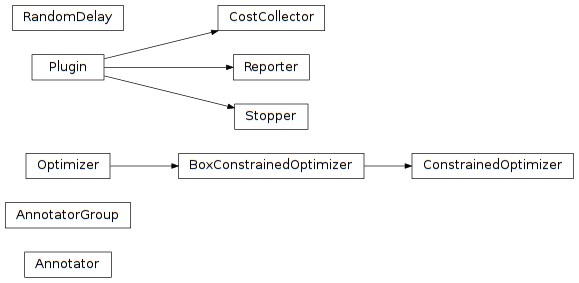Base classes for optimization algorithms and plugins (PyOPUS subsystem name: OPT)

Every optimization algorthm should be used in the following way.

1. Create the optimizer object.
2. Call the reset() method of the object to set the initial point.
3. Optionally call the check() method to check the consistency of settings.
4. Run the algorithm by calling the run() method.

The same object can be reused by calling the reset() method followed by an optional call to check() and a call to run().

class pyopus.optimizer.base.RandomDelay(obj, delayInterval)

A wrapper class for introducing a random delay into function evaluation

Objects of this class are callable. On call they evaluate the callable object given by obj with the given args. A random delay with uniform distribution specified by the delayInterval list is generated and applied before the return value from obj is returned. The two members of delayInterval list specify the lower and the upper bound on the delay. f

Example:

from pyopus.optimizer.base import RandomDelay

def f(x):
return 2*x

# Delayed evaluator with a random delay between 1 and 5 seconds
fprime=RandomDelay(f, [1.0, 5.0])

# Return f(10) without delay
print f(x)

# Return f(10) with a random delay between 1 and 5 seconds
print fprime(10)

class pyopus.optimizer.base.Plugin(quiet=False)

Base class for optimization algorithm plugins.

A plugin is a callable object with the following calling convention

plugin_object(x, ft, opt)

where x is an array representing a point in the search space and ft is the corresponding cost function value. If ft is a tuple the first member is the cost function value, the second member is an array of nonlinear constraint function values, and the third member is the vector of constraint violations. A violation of 0 means that none of the nonlinear constraints is violated. Positive/negative violations indicate a upper/lower bound on the constraint function is violated. opt is a reference to the optimization algorithm where the plugin is installed.

The plugin’s job is to produce output or update some internal structures with the data collected from x, ft, and opt.

If quiet is True the plugin supresses its output. This is useful on remote workers where the output of a plugin is often uneccessary.

reset()

Resets the plugin to its initial state.

setQuiet(quiet)

If quiet is True all further output is supressed by the plugin. To reenable it, call this method with quiet set to False.

class pyopus.optimizer.base.Reporter(onImprovement=True, onIterStep=1)

A base class for plugins used for reporting the cost function value and its details.

If onImprovement is True the cost function value is reported only when it improves on the best-yet (lowest) cost function value.

onIterStep specifies the number of calls to the reporter after which the cost function value is reported regardless of the onImprovement setting. Setting onIterStep to 0 disables this feature.

The value of the cost function at the first call to the reporter (after the last call to the reset() method) is always reported. The mechanism for detecting an improvement is simple. It compares the niter and the bestIter attributes of opt. The latter becomes equal to the former whenever a new better point is found. See the updateBest() method of the optimization algorithm for more details.

ft is a tuple for nonlinearly constrained optimizers. More details can be found in the documantation of the Plugin class.

The reporter prints the function value and the cumulative constraint violation (h).

The iteration number is obtained from the niter member of the opt object passed when the reporter is called. The best-yet value of the cost function is obtained from the f member of the opt object. This member is None at the first iteration.

class pyopus.optimizer.base.Stopper(quiet=False)

Stopper plugins are used for stopping the optimization algorithm when a particular condition is satisfied.

The actuall stopping is achieved by setting the stop member of the opt object to True.

class pyopus.optimizer.base.Annotator

Annotators produce annotations of the function value. These annotations can be used for restoring the state of local objects from remotely computed results.

Usually the annotation is produced on remote workers when the cost function is evaluated. It is the job of the optimization algorithm to send the value of ft along with the corresponding annotations from worker (where the evaluation took place) to the master where the annotation is consumed (by a call to the newResult() method of the optimization algorithm). This way the master can access all the auxiliary data which is normally produced only on the machine where the evaluation of the cost function took place.

consume(annotation)

Consume an annotation.

produce()

Produce an annotation.

class pyopus.optimizer.base.AnnotatorGroup

This object is a container holding annotators.

Adds an annotator at position index. If no index is given the annotator is appended at the end. The index of the annotator is returned.

consume(annotations)

Consumes a list of annotations.

produce()

Produces a list of annotations corresponding to annotators.

class pyopus.optimizer.base.Optimizer(function, debug=0, fstop=None, maxiter=None, nanBarrier=False, cache=False)

Base class for unconstrained optimization algorithms.

function is the cost function (Python function or any other callable object) which should be minimzied.

If debug is greater than 0, debug messages are printed at standard output.

fstop specifies the cost function value at which the algorithm is stopped. If it is None the algorithm is never stopped regardless of how low the cost function’s value gets.

maxiter specifies the number of cost function evaluations after which the algorithm is stopped. If it is None the number of cost function evaluations is unlimited.

nanBarrier specifies if NaN function values should be treated as infinite thus resulting in an extreme barrier.

cache turns on local point caching. Currently works only for algorithms that do not use remote evaluations. See the cache module for more information.

The following members are available in every object of the Optimizer class

• ndim - dimension of the problem. Updated when the reset() method is called.
• niter - the consecutive number of cost function evaluation.
• x - the argument to the cost function resulting in the best-yet (lowest) value.
• f - the best-yet value of the cost function.
• bestIter - the iteration in which the best-yet value of the cost function was found.
• bestAnnotations - a list of annotations produced by the installed annotators for the best-yet value of the cost function.
• stop - boolean flag indicating that the algorithm should stop.
• annotations - a list of annotations produced by the installed annotators for the last evaluated cost function value
• annGrp - AnnotatorGroup object that holds the installed annotators
• plugins - a list of installed plugin objects

Plugin objects are called at every cost function evaluation or whenever a remotely evaluated cost function value is registered by the newResult() method.

Values of x and related members are arrays.

check()

Checks the optimization algorithm’s settings and raises an exception if something is wrong.

fun(x, count=True)

Evaluates the cost function at x (array). If count is True the newResult() method is invoked with x, the obtained cost function value, and annotations argument set to None. This means that the result is registered (best-yet point information are updated), the plugins are calls and the annotators are invoked to produce annotations.

Use False for count if you need to evaluate the cost function for debugging purposes.

Returns the value of the cost function at x.

getEvaluator(x)

Returns a tuple holding the evaluator function and its positional arguments that evaluate the problem at x. This tuple can be sent to a remote computing node and evaluation is invoked using:

# Tuple t holds the function and its arguments
func,args=t
retval=func(*args)
# Send retval back to the master


The first positional argument is the point to be evaluated.

The evaluator returns a tuple of the form (f,annotations).

installPlugin(plugin)

Installs a plugin object or an annotator in the plugins list and/or annotators list

Returns two indices. The first is the index of the installed annotator while the second is the index of the instaleld plugin.

If teh object is not an annotator, the first index is None. Similarly, if the object is not a plugin the second index is None.

newResult(x, f, annotations=None)

Registers the cost function value f obtained at point x with annotations list given by annotations.

Increases the niter member to reflect the iteration number of the point being registered and updates the f, x, and bestIter members.

If the annotations argument is given, it must be a list with as many members as there are annotator objects installed in the optimizer. The annotations list is stored in the annotations member. If f improves the best-yet value annotations are also stored in the bestAnnotations member. The annotations are consumed by calling the consume() method of the annotators.

Finally it is checked if the best-yet value of cost function is below fstop or the number of iterations exceeded maxiter. If any of these two conditions is satisfied, the algorithm is stopped by setting the stop member to True.

reset(x0)

Puts the optimizer in its initial state and sets the initial point to be the 1-dimensional array or list x0. The length of the array becomes the dimension of the optimization problem (ndim member).

run()

Runs the optimization algorithm.

updateBest(x, f)

Updates best yet function value. Returns True if an update takes place.

class pyopus.optimizer.base.BoxConstrainedOptimizer(function, xlo=None, xhi=None, debug=0, fstop=None, maxiter=None, nanBarrier=False, extremeBarrierBox=False, cache=False)

Box-constrained optimizer class

xlo and xhi are 1-dimensional arrays or lists holding the lower and the upper bounds on the components of x. Some algorithms allow the components of xlo to be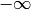and the components of xhi to be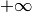.

If extremeBarrierBox is set to True the fun() method returns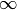if the supplied point violates the box constraints.

See the Optimizer class for more information.

bound(x)

Fixes components of x so that the bounds are enforced. If a component is below lower bound it is set to the lower bound. If a component is above upper bound it is set to the upper bound.

check()

Checks the optimization algorithm’s settings and raises an exception if something is wrong.

denormalize(y)

Returns a denormalized point x corresponding to y. Components of x are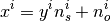fun(x, count=True)

Evaluates the cost function at x (array). If count is True the newResult() method is invoked with x, the obtained cost function value, and annotations argument set to None. This means that the result is registered (best-yet point information are updated) and the plugins are called to produce annotations.

Use False for count if you need to evaluate the cost function for debugging purposes.

Returns the value of the cost function at x.

getEvaluator(x)

Returns the evaluator function and its positional arguments that evaluate the problem at x.

The first positional argument is the point to be evaluated.

The evaluator returns a tuple of the form (f,annotations).

normDist(x, y)

Calculates normalized distance between x and y.

Normalized distance is calculated as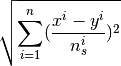normalize(x)

Returnes a normalized point y corresponding to x. Components of y are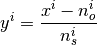If both bounds are finite, the result is within the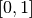interval.

reset(x0)

Puts the optimizer in its initial state and sets the initial point to be the 1-dimensional array or list x0. The length of the array becomes the dimension of the optimization problem (ndim member). The shape of x must match that of xlo and xhi.

Normalization origin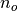and scaling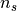are calculated from the values of xlo, xhi, and intial point x0:

• If a lower bound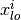is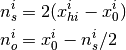• If an upper bound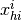is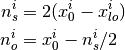• If both lower and upper bound are infinite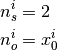• If bouth bounds are finite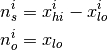class pyopus.optimizer.base.ConstrainedOptimizer(function, xlo=None, xhi=None, constraints=None, clo=None, chi=None, fc=None, debug=0, fstop=None, maxiter=None, nanBarrier=False, extremeBarrierBox=False, cache=False)

Constrained optimizer class

xlo and xhi are 1-dimensional arrays or lists holding the lower and upper bounds on the components of x. Some algorithms allow the components of xlo to beand the components of xhi to be.

constraints is a function that returns an array holding the values of the general nonlinear constraints.

clo and chi are vectors of lower and upper bounds on the constraint functions in constraints. Nonlinear constraints are of the form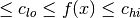.

fc is a function that simultaneously evaluates the function and the constraints. When it is given, function and constraints must be None.

See the BoxConstrainedOptimizer class for more information.

aggregateConstraintViolation(c, useL2squared=False)

Computes the aggregate constraint violation. If no nonlinear constraints are violated this value is 0. Otherwise it is greater than zero.

c is the vector of constraint violations returned by the constraintViolation() method.

if useL2squared is True the L2 norm is used for computing the aggregate violation. Otherwise L1 norm is used.

check()

Checks the optimization algorithm’s settings and raises an exception if something is wrong.

constraintViolation(c)

Returns constraint violation vector. Negative values correspond to lower bound violation while positive values correspond to upper bound violation.

Returns 0 if c is zero-length.

fun(x, count=True)

The use of this function is not allowed in constrained optimization.

funcon(x, count=True)

Evaluates the cost function and the nonlinear constraints at x.

If count is True the newResult() method is invoked with x, the obtained cost function value, the nonlinear constraint values, and the annotations argument set to None. This means that the result is registered (best-yet point information are updated) and the plugins are called to produce annotations.

Use False for count if you need to evaluate the cost function and the constraints for debugging purposes.

Returns the value of the cost function at x adjusted for extreme barrier (i.e. Inf when box constraints are violated or the function value is Nan) and a vector of constraint function values.

getEvaluator(x)

Returns the evaluator function and its positional arguments that evaluate the problem at x.

The first positional argument is the point to be evaluated.

The evaluator returns a tuple of the form (f,c,annotations).

newResult(x, f, c, annotations=None)

Registers the cost function value f and constraints values c obtained at point x with annotations list given by annotations.

Increases the niter member to reflect the iteration number of the point being registered and updates the f, x, c, and bestIter members.

See the newResult() method of the Optimizer class for more information.

reset(x0)

Puts the optimizer in its initial state and sets the initial point to be the 1-dimensional array or list x0. The length of the array becomes the dimension of the optimization problem (ndim member). The shape of x must match that of xlo and xhi and x must be within the bounds specified by xlo and xhi.

See the reset() method of BoxConstrainedOptimizer class for more information.

updateBest(x, f, c)

Updates best yet function and constraint values.

Calculates cumulative squared constraint violation (h). It is 0 if there are no constraints.

If h<hbest, replace best point. If h==hbest and f<fbest, replace best point.

Returns True if an update takes place.

pyopus.optimizer.base.UCEvaluator(x, f, annGrp, nanBarrier)

Evaluator for unconstrained optimization.

Returns the function value and the annotations.

This function should be used on a remote computing node for evaluating the function. Its return value should be sent to the master that invoked the evaluation.

The arguments for this function are generated by the optimizer. See the getEvaluator() method.

The evaluator and its arguments are the minimum of things needed for a remote evaluation.

By using this function one can avoid pickling and sending the whole optimizer object with all of its auxiliary data. Instead one can just pickle and send the bare minimum needed for a remote evaluation.

pyopus.optimizer.base.BCEvaluator(x, xlo, xhi, f, annGrp, extremeBarrierBox, nanBarrier)

Evaluator for box-constrained optimization.

Returns the function value and the annotations.

The arguments are generated by the optimizer. See the getEvaluator() method.

The evaluator and its arguments are the minimum of things needed for a remote evaluation.

See the UCEvaluator() function for more information.

pyopus.optimizer.base.CEvaluator(x, xlo, xhi, f, fc, c, annGrp, extremeBarrierBox, nanBarrier)

Evaluator for constrained optimization.

Returns the function value, the constraints values, and the annotations.

The arguments are generated by the optimizer. See the getEvaluator() method.

The evaluator and its arguments are the minimum of things needed for remote evaluation.

See the UCEvaluator() function for more information.

pyopus.optimizer.base.normalizer(x, origin, scale)

Normalizer for bound constrained optimization.

pyopus.optimizer.base.denormalizer(y, origin, scale)

Denormalizer for bound constrained optimization.

class pyopus.optimizer.base.CostCollector(chunkSize=100)

A subclass of the Plugin iterative algorithm plugin class. This is a callable object invoked at every iteration of the algorithm. It collects the input parameter vector (n components) and the aggregate function value.

Let niter denote the number of stored iterations. The input parameter values are stored in the xval member which is an array of shape (niter, n) while the aggregate function values are stored in the fval member (array with shape (niter)). If the algorithm supplies constraint violations they are stored in the hval member. Otherwise this member is set to None.

Some iterative algorithms do not evaluate iterations sequentially. Such algorithms denote the iteration number with the index member. If the index member is not present in the iterative algorithm object the internal iteration counter of the CostCollector is used.

The first index in the xval, fval, and hval arrays is the iteration index. If iterations are not performed sequentially these two arrays may contain gaps where no valid input parameter or aggregate function value is found. The gaps are denoted by the valid array (of shape (niter)) where zeros denote a gap.

xval, fval, hval, and valid arrays are resized in chunks of size chunkSize.

finalize()

Removes the space beyond the recorded iteration with highest iteration number. This space was reserved for the last chunk, but the highest recorded iteration may not be the one recorded at the end of the chunk.

Must be called after the iterative algorithm is stopped.

reset()

Clears the xval, fval, hval, and valid members.

#### Previous topic

4. pyopus.optimizer — Optimization algorithms

#### Next topic

4.2. pyopus.optimizer.cache — Caching of function and constraint values, and annotations